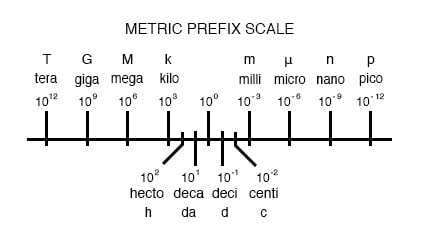Vol. Direct Current (DC)
Chapter 4 Scientific Notation And Metric Prefixes

# Metric Notation

The metric system, besides being a collection of measurement units for all sorts of physical quantities, is structured around the concept of scientific notation. The primary difference is that the powers-of-ten are represented with alphabetical prefixes instead of by literal powers-of-ten.

The following number line shows some of the more common prefixes and their respective powers-of-ten:Looking at this scale, we can see that 2.5 Gigabytes would mean 2.5 x 109 bytes, or 2.5 billion bytes. Likewise, 3.21 picoamps would mean 3.21 x 10-12 amps, or 3.21 1/trillionths of an amp.

Other metric prefixes exist to symbolize powers of ten for extremely small and extremely large multipliers. On the extremely small end of the spectrum, femto (f) = 10-15, atto (a) = 10-18, zepto (z) = 10-21, and yocto(y) = 10-24. On the extremely large end of the spectrum, Peta (P) = 1015, Exa (E) = 1018, Zetta (Z) = 1021, and Yotta (Y) = 1024.

Because the major prefixes in the metric system refer to powers of 10 that are multiples of 3 (from “kilo” on up, and from “milli” on down), metric notation differs from regular scientific notation in that the mantissa can be anywhere between 1 and 999, depending on which prefix is chosen.

For example, if a laboratory sample weighs 0.000267 grams, scientific notation and metric notation would express it differently:

2.67 x 10-4 grams (scientific notation)

267 µgrams (metric notation)

The same figure may also be expressed as 0.267 milligrams (0.267 mg), although it is usually more common to see the significant digits represented as a figure greater than 1.

In recent years a new style of metric notation for electric quantities has emerged which seeks to avoid the use of the decimal point. Since decimal points (”.”) are easily misread and/or “lost” due to poor print quality, quantities such as 4.7 k may be mistaken for 47 k.

The new notation replaces the decimal point with the metric prefix character, so that “4.7 k” is printed instead as “4k7”. Our last figure from the prior example, “0.267 m”, would be expressed in the new notation as “0m267”.

REVIEW:

• The metric system of notation uses alphabetical prefixes to represent certain powers-of-ten instead of the lengthier scientific notation.

RELATED WORKSHEETS:

#### Lessons in Electric Circuits

##### Volumes »
Published under the terms and conditions of the Design Science License• B
Bruce1956 April 26, 2020

More of the same here, although on this page, some of the exponents are expressed correctly.  First example:

“On the extremely large end of the spectrum, Peta (P) = 1015, Exa (E) = 1018, Zetta (Z) = 1021, and Yotta (Y) = 1024.”  Should be:

“On the extremely large end of the spectrum, Peta (P) = 10¹⁵, Exa (E) = 10¹⁸, Zetta (Z) = 10²¹, and Yotta (Y) = 10²⁴.

Like.
• B
Bruce1956 April 26, 2020

In comparing with the .pdf version, the .pdf has none of these problems with a lack of superscripts for exponents.  I copied the correct version from the .pdf and pasted it here as an experiment, and the formatting was lost.  I don’t know of an efficient way to copy and paste from a .pdf without losing the formatting,  I could do it manually as I did below, but it would be time consuming.  If you know of a better method, please let me know.  Maybe converting the .pdf to a normal (but still properly formatted) text, then pasting that to the page?  Or maybe use an editor that automatically converts .pdf to .html?

Like.
• B
Bruce1956 April 26, 2020

In any case, the easiest solution for me will be to download the .pdf and use that, rather than the individual pages, as I continue to read the book.  However, I’ll fix this if you’d like me to, as I’m retired and have the time to contribute.  I would still appreciate guidance on method of correction and will need to know the file type of corrected text you’d like and how to submit it.  Thanks!

Like.